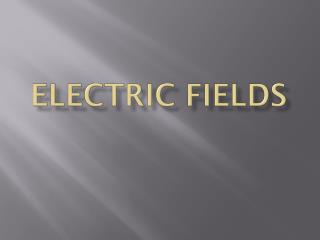DownloadDownload PresentationELECTRIC FIELDS

# ELECTRIC FIELDS

Download Presentation## ELECTRIC FIELDS

- - - - - - - - - - - - - - - - - - - - - - - - - - - E N D - - - - - - - - - - - - - - - - - - - - - - - - - - -
##### Presentation Transcript

1. ELECTRIC FIELDS

2. I. Electric Field • Assume you have a point charge somewhere in space. • If you place a tiny point charge (q0), into that space, the electric field will apply a force to that test charge. q0 +

3. II. Electric Field Strength • FORMULA • F is the force on the test charge. • Unit of Electric Field is a N/C E = F/q0 or E= kq/r2

4. III. Other Electric Field Formulas • E = V/d • As distance increases from the Charge, the potential, or work decreases. • V= kq/r • The closer you get to a charge, the greater the potential • The bigger the charge the greater the potential

5. III. Electric Field Lines • Always go from positive to negative. • OR, • Positive away • Negative toward • EXAMPLES • Hey, it is easier to draw them by hand

6. PROBLEMS • What is the Electric Field strength of a point charge if .0002 N push an electron? • What is the Field strength .02 m from 2.0 x 1015 protons?